# ICSE Solutions for Class 10 Physics – Force, Work, Power and Energy

## ICSE Solutions for Class 10 Physics – Force, Work, Power and Energy

APlusTopper.com provides ICSE Solutions for Class 10 Physics Chapter 1 Force, Work, Power and Energy for ICSE Board Examinations. We provide step by step Solutions for ICSE Physics Class 10 Solutions Pdf. You can download the Class 10 Physics ICSE Textbook Solutions with Free PDF download option.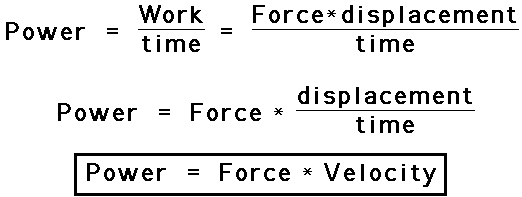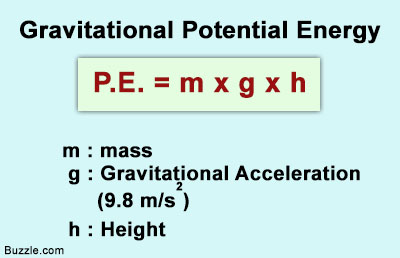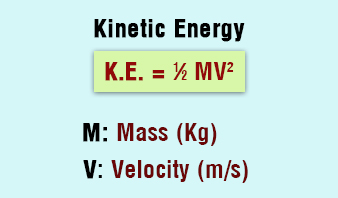Question 1: Define force. Is force a scalar or a vector quantity?
Answer: A force is that cause which changes or tends to change the state of rest or of uniform motion of a body. Force is a vector quantity.

Question 2: State and define the unit of force in the S.I. system.
Answer: The unit of force in S. I. system is Newton (N). Newton is defined as the force which, when applied on a body of mass 1 kg produces in it an acceleration of 1 ms2.

Question 3: Define one newton. Write the relation between S. I. unit and C.G.S. unit of force.
Answer: One Newton: If a body of mass 1 kg moves with an acceleration of 1 m/s2 then force acting on the body is said to be one Newton. 1N = 105 Dyne.
Relation between S. I. and CGS unit of force is 1 Newton = 1 kg × 1 ms-2.

Question 4: Define 1 kgf., How is it related to the S.I. unit of force?
Answer: 1 kgf: It is the force due to gravity on a mass of 1kg. (kilogram), 1 kgf = 9.8 N.

Question 5: (i) Where is the centre of gravity of a uniform ring situated?
(ii) ‘The position of the centre of gravity of a body remains unchanged even when the body is deformed.’ State whether the statement is true or false.
Answer: (i) Centre of gravity of a uniform ring is at its centre.
(ii) False.

Question 6: Which of the following remains constant in uniform circular motion, Speed or Velocity or both?
Answer: In uniform circular motion speed remains constant.

Question 7: Define 1 Dyne.
Answer: 1 dyne is that force which when acting on a body of mass 1 gram, produces an acceleration of 1 cm s-2 in it.
1 dyne = 1 g × 1 cm s-2.

Question 8: Define contact forces.
Answer: The forces which are applied on a body through a connector, are called contact forces. Forces like Frictional force, Mechanical force etc., are the forces of contact.

Question 9: Define non-contact forces.
Answer: The forces which act on a body without the help of any connector, are called non-contact forces or forces of distance. Gravitational force, Mechanical force etc., are non-contact forces.

Question 10: State the energy changes in the following devices while in use
(i) A loud speaker (ii) A glowing electric bulb
Answer: (i) Loud speaker: Electric energy to sound.
(ii) Glowing electric bulb: Electric to heat energy to light energy.

Question 11: Give any two effects of a force on a non-rigid body.
Answer: Two effects of a force on a non-rigid body are:
(i) Force can change the state of rest or motion of the body
(ii) Force can change the size or shape of the body.

Question 12: Where does the position of centre of gravity lie for
(i) a circular lamina (ii) a triangular lamina
Answer: (i) Circular lamina – Centre of the lamina
(ii) Triangular lamina – Point of intersection of medians.

Question 13: What is Gravitational force? Give gravitational units of force.
Answer: Earth exerts a force of attraction on each object. This force is due to gravity and its magnitude is directly proportional to the mass of the object. This force is known as gravitational force and its units are gravitational units.
In MKS system the gravitational unit of force is kilogramme force i.e., kgf. In CGS system it is gramme force, gf.

Question 14: What is the work done by the gravitational force on the moon that revolves around the earth?
Answer: The moon is revolving around the earth. In this process, the work done by the gravitational force is zero because the displacement of the moon is at right angle to the gravitational force.

Question 15: A coolie, with a load of 30 kg on his head, walks on the platform. If he walks a distance of 60 m, what is the work done by him?
Answer: The work done by the coolie is zero because there is no displacement of’ the load in the direction of force, which he is applying vertically upwards.

Question 16: A bullet fired against a window glass pane makes a hole in it without any cracks while a stone striking the same glass pane smashes it; explain with reason.
Answer: When the bullet strikes the glass, the part of the glass coming in contact with the bullet, immediately shares the large velocity of the bullet and makes a hole in it while the rest of the glass pane, due to inertia of rest, remains at rest and so is not smashed. But when a stone strikes the same glass pan, the surrounding part of the glass gets time to share the comparatively low velocity of stone and so it is smashed.

Question 17: State one factor on which the magnitude of a non-contact force depends. How does magnitude of a non-contact force change along distance?
Answer: The magnitude of a non-contact force depends on distance. If magnitude of a non-contact force increasing then distance decreasing.

Question 18: Under what condition will a set of gears produce
(i) a gain in speed (ii) a gain in torque.
Answer: (i) Gain in speed: When number of teeth in driving wheel is more than number of teeth in driven wheel.
(ii) Gain in torque: When number of teeth in driven gear is more than number of teeth in driving gear.

Question 19: Name the force required for uniform circular motion. State its direction.
Answer: Force required for uniform circular motion is centripetal force. It is directed towards its centre i.e., centre of the circle.
A force is applied on (i) a non-rigid body and (ii) a rigid body. How does the effect of the force differ in the above two cases?

Question 20: A force is applied on (i) a non-rigid body and (ii) a rigid body. How does the effect of the force differ in the above two cases?
Answer: When force is applied on a non-rigid body it undergoes deformation i.e. change in size, shape and position where as a rigid body doesn’t undergo change in shape, position and size.

Question 21: How does the distance of separation between two bodies affect the magnitude of the non-contact force between them?
Answer: Non-contact force decreases with the increase in separation.

Question 22: In what way does an ‘Ideal machine’ differ from a ‘Practical machine’?
Answer: Ideal machine has 100% efficiency i.e., work done on the machine = work done by the machine.

Question 23: Can a simple machine act as a force multiplier and a speed multiplier at the same time?

Question 24: State two factors on which moment of force about a point depends.
Answer: Moment of force depends on two factors:
(i) Magnitude of force applied.
(ii) Distance of line of action of the force from the axis of rotation.

Question 25: State the units of moment of force.
Answer: In CGS system, unit of moment of a force is Dyne-cm and in MKS system, unit of moment of a force is Newton-metre. The gravitational units in CGS and MKS system of measurement are gf cm and kg m respectively.

Question 26: State the principle of moments.
Answer: When number of forces acting on a body, keep it in equilibrium, then the sum total of clockwise moments about any turning point is equal to sum total of anticlockwise moments about the point.

Question 27: State when moment of force is positive and when it is negative.
Answer: If couple has a tendency to rotate the body in anti-clockwise direction then its moment is taken positive and if the tendency of rotation is clockwise then the moment is negative.

Question 28: Give the units of couple.
Answer: In MKS system, the unit of couple are Nm and kgf m.
In CGS system, the units of couple are Dyne cm and gf cm.

Question 29: Two similar vehicles are moving with same velocity on the road, such that one of thus is loaded and the other one is empty which of the two vehicles will require larger force to stop?
Answer: A large force is required to stop the loaded vehicle. It is because loaded vehicle has greater momentum than the empty vehicle as the mass of loaded vehicle is more than that of the empty one. So, it requires more force to stop.

Question 30: What is meant by the term ‘moment of force’? If the moment of force is assigned a negative sign then will the turning tendency of the force be clockwise or anti clockwise?
Answer: It is equal to the product of the magnitude of the force and the perpendicular distance of the line of action of force from the axis of rotation. If moment of force is assigned a negative value, it means clockwise tendency of force.

Question 31: Define Uniform circular motion.
Answer: When a particle moves with a constant speed in a circular path, its motion is said to be the uniform circular motion.

Question 32: Define Translational motion.
Answer: When a force acts on a rigid body which is free to move, the body starts moving in a straight line in the direction of force. This is called translational motion.

Question 33: Define Centripetal force.
Answer: At each of circular path, the particle, instead of moving straight continuously, turns towards the centre. Therefore, the motion in circular path is under the action of a force which called the centripetal force.

Question 34: Define the term momentum.
Answer: The momentum of a body is the product of the mass of the body and its velocity, i.e. p = mv.

Question 35: How is force related to the momentum of a body?
Answer: Force is equal to the rate of change of momentum.

Question 36: State the condition when the change in momentum of a body depends only on the change in its velocity.
Answer: If mass of the body m remains constant then the change in momentum of the body depends only on the change in its velocity.

Question 37: With reference to their direction of action, how does a centripetal force differ from a centrifugal force?
Answer: Direction of centripetal force is towards the centre of the circle whereas centrifugal force is opposite to centripetal force.

Question 38: Can the CG be situated outside the material of the body? Give an example.
Answer: The position of CG of a body depends on the distribution of mass in the body. It can be situated outside or inside the material of the body.
For example: The CG of a wire is at its mid-point but CG of a ring lies at the centre where there is no material.

Question 39: Explain why It is easier to knock down a boy who is standing on one foot than one who is standing on two.
Answer: It is easier to knock down a boy who is standing on one foot than one who is standing on two because boy standing on both feet has a larger base area and hence it has more stable equilibrium than a boy standing on one foot.

Question 40: Explain why One leans forward while climbing up a hill.
Answer: A person going uphill has to bend forward but while coming down has to bent backwards, this is to keep the CG at the centre of the body and between his feet.

Question 41: Explain why a tight rope-walker, often holds a long pole in his hands when in action.
Answer: A tight rope-walker carries a long stick in his hand to maintain the CG such that the vertical line passes through the rope.

Question 42: Explain why a ship loaded with light goods is more liable to be over turned than the one loaded with heavy goods.
Answer: A ship loaded with light goods is more liable to be over turned than the one loaded with heavy goods. This is because the CG in the former case is high and so a slight displacement due to water current may cause the vertical line passing through the CG to fall outside the base, thereby overturning the ship.

Question 43: Explain why Standing passengers are not allowed on the upper deck of a double decker bus.
Answer: The centre of gravity is raised and on an inclined road the bus may topple.

Question 44: Explain when we carry a weight on one hand, we bend on the other side.
Answer: This is to let our CG fall within the base of our support.

Question 45: What is the weight of a body placed at the centre of the earth?
Answer: The weight of a body placed at the centre of the earth is zero as
g = 0
∴ W = mg
= 0

Question 46: What do you understand by work? Is work a scalar or a vector quantity?
Answer: Work is said to be done when a force displaces a body through some distance, in its own direction, i.e.,          Work = Force × Displacement
W = F × d
Work is a scalar quantity.

Question 47: In the following cases write yes, if the work is being done and no, if no work is being done.
(i) A man trying to push a wall, (ii) A man moving on the rough ground.
(iii) A coolie standing with a heavy load on his head, (iv) A boy climbing up a staircase.
Answer: (i) No. (ii) Yes. (iii) No. (iv) Yes.

Question 48: State two conditions when the work done by a force acting on a body is zero.
Answer: (i) When there is no displacement by the force in the body.
(ii) When the displacement is normal to the direction of force.

Question 49: (i) Define power.
(ii) Is power a scalar or vector quantity?
Answer: (i) Power is defined as the rate of doing work or work done per second,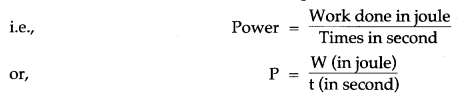(ii) Power is a scalar quantity.

Question 50: State the condition when the work done by force is (i) positive and (ii) negative.
Answer: (i) Work done by a force is positive when the displacement is in the direction of the force.
(ii) Work done by a force is negative when the displacement is in the direction opposite to the force.

Question 51: A force F when acts on a body, displaces it by a distance d in a direction at an angle θ with the direction of force. Write down the expression for the work done by the force.
Answer: Work done by the force, W = Fd cos θ.

Question 52: Can two agents, doing the same work, have different power?
Answer: Yes, since power = work/time the two agents can have different powers if they take different amounts of time to do the same work.

Question 53: When does a force do work? What is the work done by the moon when it revolves around the earth?
Answer: Work is said to be done if a body undergoes displacement due to applied force.
Work done = Force × Displacement
Work done is zero by the moon, as there is no displacement since it is moving in a circular path.

Question 54: State the amount of work done by an object when it moves in a circular path for one complete rotation. Give a reason to justify your answer.
Answer: Amount of work done is equal to zero.
Work is said to be done only when there is displacement produced. In case of a body moving in a circular path, the body comes to its original place, therefore, there is no displacement and hence work is zero.

Question 55: State two conditions for work to be done.
(i) Application of-force on the body.
(ii) Displacement of the body in the direction of force.

Question 56: Why is less effort needed to lift a load over an inclined plane as compared to lifting the load directly?
Answer: Less effort is required because for a given height ‘h’ as longer is the length ‘l’ of the inclined plane, smaller is the angle of inclination therefore, greater is the mechanical advantage, so lesser will be the effort required. As we know

Question 57: What is the S.I. unit of work? Define it.
Answer: The S.I. unit of work is newton-metre or joule (J).
1 Joule = 1 N × 1 m.
1 J of work is said to be done by a force of 1 N if it displaces a body by 1 m in the direction of force.

Question 58: State S.I. unit of power and define it.
Answer: S.I. unit of power is J/s or Js-1 (or watt W). The power is said to be 1 watt when 1 J of work is done in 1 sec.

Question 59: Justify the statement. “Power can be expressed as the product of force and velocity.”(Since rate of change of displacement is velocity).

Question 60: State larger units of power.
Answer: The larger units of power are:
1 Kilowatt = 1000 watt 103 W
1 Megawatt = 1000,000 W = 106 W.

Question 61: State the practical unit mainly used in mechanical engineering and write down its equivalent in watt.
Answer: The practical unit of power used in mechanical engineering is ‘Horse Power’ (H.P,)
1 H.P. = 746 W = 0.746 KW.

Question 62: What happen to the power if the time for doing a work is reduced from t second to t/10 second?
Answer: The power becomes 10 times of its initial value. This is because power = work/time. Thus, power ∝ 1/time for a given work. Reducing the time by a factor of 10 thus increases the power 10 times.

Question 63: What do you understand by the term energy? Is energy a scalar or vector? How is energy possessed by a body measured?
Answer: Energy is defined as the capacity of a body to do work. Energy is a scalar quantity. The energy possessed by a body is measured by the amount of work done by a body while changing its state of rest or state of motion.

Question 64: State the S.I. and C.G.S. units of energy. How are they related?
Answer: The S.I. unit of energy is joule (J) and C.G.S. unit is erg.
1J = 107 erg.

Question 65: Write five forms of energy.
Answer: (i) Mechanical energy (ii) Chemical energy (iii) Solar energy
(iv) Heat energy (v) Light energy.

Question 66: What do you understand by mechanical energy? What are the different forms of mechanical energy?
Answer: The energy possessed by body due to its state or its motion is called mechanical energy. Mechanical energy is of two forms: (i) potential energy, and (ii) kinetic energy.

Question 67: What do you understand by the potential energy of the body?
Answer: The potential energy of a body is the energy possessed by body by virture of its state or position.

Question 68: What do you understand by the kinetic energy of the body? Give three examples of bodies possessing kinetic energy.
Answer: The kinetic energy of a body is the energy possessed by body by virtue of its state of motion,
(i) A moving bullet (ii) A flowing river (iii) Wind.

Question 69: How is kinetic energy possessed by a moving body measured?
Answer: The kinetic energy possessed by a moving body is measured by the amount of work done by an opposing force in bringing the body to rest from its present state of motion.

Question 70: A man climbs a slope and another walks same distance on level road. Which of the two expends more energy and why?
Answer: The man moving along the slope expends more energy. It is because, while moving along slope, he gains height and hence has extra potential energy.

Question 71: Is it possible that a body may possess energy even when it is not in motion?
Answer: Yes, the body may possess potential energy.

Question 72: What kind of energy is possessed in the situations when?
(i) A cocked up spring of air gun. (ii) A stone lying on the top of roof.
(iii) A fish moving in water. (iv) A horse running along a level road,
(v) Water stored in dams. (vi) An electron spinning around nucleus.
(vii) A shooting arrow. (viii) A stone, in a strecthed catapult.
Answer: (i) P.E.      (ii) P.E.      (iii) K.E.
(iv) K.E.      (v) P.E.      (vi) K.E.
(vii) K.E.     (viii) P.E.

Question 73: Relate 1 KWh (kilowatt hour) with joule.
Answer: 1 KWh = 1 KW × 1 h = 1000 W × 3600s = 36,00,000 J.

Question 74: Obtain an expression for the potential energy of a body of mass m at a height h above the ground.
Answer: Force due to gravity on the body F = mg
Work done in lifting the body against the force of gravity to a height h above the ground
W = F × d = mg × h = mgh
Thus, potential energy of body = mgh.

Question 75: When an arrow is shot from its bow, it has kinetic energy. From where does it get the kinetic energy?
Answer: A stretched bow possessed potential energy on account of a change in its shape. To shoot an arrow, the bow is released. The potential energy of the bow is converted into the kinetic energy of the arrow.

Question 76: What happens to the K.E. when (i) the mass of the body is doubled at constant velocity and (ii) the velocity of the body is doubled at constant mass?
Answer: We have, K.E. = 1/2 mv2
Hence,
(i) On doubling the mass, at constant velocity, the K.E. becomes double of its original value.
(ii) On doubling the velocity, at constant mass, the K.E. becomes (2)2 i.e., 4 times its original value.

Question 77: Write the type of energy possessed in the following cases:
(i) A bent bow (ii) A falling apple
(iii) A wound up spring (iv) A moving cricket ball.
Answer: (i) A bent bow: Potential Energy
(ii) A falling apple: Kinetic Energy
(iii) A wound up spring: Potential Energy
(iv) A moving cricket ball: Kinetic Energy.

Question 78: Give three examples of bodies possessing potential energy.
Answer: (i) An object kept at a height above the ground.
(ii) A compressed spring, and
(iii) A stretched catapult.

Question 79: What is dissipation of energy?
Answer: In transforming the mechanical energy if a part of it gets converted into another form of energy which cannot be utilised for useful work, this loss of energy is called the dissipation of energy.

Question 80: State the energy changes which takes place in Electricity is obtained from solar energy.
Answer: Heat energy from sun is converted into electricity.

Question 81: What is the main energy transformation that occurs in:
(i) Photosynthesis in green leaves;
(ii) Charging of a battery.
Answer: (i) Light energy of chemical energy.
(ii) Electric energy to chemical energy.

Question 82: A man climbs a slope and another walks same distance on level road. Which of the two expends more energy and why?
Answer: The man moving along the slope expends more energy. It is because, while moving along slope, he gains height and hence has extra potential energy.

Question 83: State the law of conservation of energy.
Answer: According to law of conservation of mechanical energy, the sum of kinetic energy and potential energy always remains constant.

Question 84: Name the two groups in which various sources of energy are classified?
Answer: Renewable and Non-renewable sources of energy.

Question 85: Give two examples each of renewable and non-renewable sources of energy?
Answer: Renewable sources of energy: Solar energy and wind energy.
Non-renewable sources of energy: coal and oil.

Question 86: Why using wood as fuel is not advisable although wood is a renewable source of energy?
Answer: It is not advisable to use wood as fuel even though it can be replenished because burning of
wood produces smoke that causes pollution which results in environmental imbalance.

Question 87: Name a device in which solar energy is converted into electricity state its two uses.
Answer: Solar cell. Its two uses are:
(i) All artificial satellites and space probes mainly depend upon electricity generated by solar cells.
(ii) Solar cells are used for lighting, operating water pumps, for running radio and television sets in remote areas and traffic signals etc.

Question 88: Name the material commonly used for manufacturing of solar cells.
Answer: Silicon and Gallium. Solar cell produces d.c. current. State whether a solar cell produces a.c. or d.c. current.

Question 89: Name two places in India where electricity is generated from nuclear power plants.
(ii) At Narora, in Uttar Pradesh.

Question 90: What should be the characteristic of a source of energy?
Answer: A good source of energy should have the following characteristics:
(i) It should do a large amount of work per unit mass or volume.
(ii) It should have high calorific value.
(iii) It should be easily accessible over a long period of time.
(iv) It should be economical and easy to store and transport.
(v) It should be Safe and convenient to use.

Question 91: What is hydro-energy? Explain the principle of generating electricity from hydro-energy.
Answer: The energy possessed by the flowing water is called hydro-energy.
Principle: It works on the principle of conversion of kinetic energy of flowing water into electrical energy. When the stored water is allowed to fall from the top of dam on the blades of water-turbine, the potential energy of stored water is converted into kinetic energy which rotates the water turbine rapidly which in turn rotates the armature coil of generator and generates electricity.

Question 92: How much hydro-electric power is generated in India?
Answer: At present, 23% of total electricity is generated in India using hydro-energy.

Question 93: State two advantages and two disadvantages of using hydro-energy for producing electricity.
(i) When the electricity is generated, no greenhouse gases are made.
(ii) It is an inexhaustible source of energy.
(i) The dam is expensive to build.
(ii) By building a dam, the nearby area has to be flooded and this could affect nearby wildlife and Plants.

Question 94: What is wind energy? Explain the principle of working of windmill for generating electricity.
Answer: The blowing wind has kinetic energy is called wind energy.
Principle: It works on the principle of conversion of kinetic energy of blowing wind into electrical energy. When the blowing wind strikes across the blades of a windmill it exerts a force which rotates its blade. The turning of the blades would simultaneously cause the shaft to turn, which is connected to a generator. This makes the generator produce electricity.

Question 95: State two advantages and disadvantage of using wind energy for producing electricity.
(i) Wind is free and will not run not (renewable sources).
(ii) Wind energy does not create greenhouse gases.
(i) The wind farms can be established only at places near the coastal areas where wind blows around the year steadily with a speed not less than 15 Km h-1.
(ii) The establishment of wind farm is expensive.

Question 96: State two advantages and disadvantage of using nuclear energy for producing electricity.
(i) A very small amount of nuclear fuel produces huge amount of energy.
(ii) Nuclear fuel does not contribute harmful greenhouse gases to the atmosphere on burning.
(i) The waste that is produced when using nuclear fuel is radioactive and very harmful. It needs to be disposed of carefully.
(ii) World uranium supplies may run out in about 50 year.

Question 97: State two ways for the judicious use of energy.
Answer: (i) The wastage of energy should be avoided.
(ii) The excessive use of non-renewable resources such as coal, petroleum and natural gas should be reduced.

Question 98: Explain, why submarines are able to dive under water as well as sail on the surface of water?
Answer: Submarines are provided with ballast tanks. When required to dive, the water is allowed to enter in the tanks. And when required to sail, the water is forced out by compressed air.
Work is a scalar quantity.

Question 99: State the principle of conservation of energy.
Answer: Principle of conservation of energy: It states that energy can neither be created nor be destroyed but can be transferred from one form to another form.

Question 100: Distinguish between work and power.
Answer: Work does not depend on time, while the power depends on time. Power is the work done in unit time.

Question 101: State the energy changes which takes place when:

1. A body released from rest from the top of a building.
2. A body thrown vertically upwards from the ground.
3. In a heat engine.
4. A bulb glows, when torch light is switched on.
5. A car moves up a hill.
6. A toy car spring is wound and car is made to run on level floor.
7. Water stored in dams rotates turbine connected to a dynamo.
8. An air gun is loaded and then fired.
9. A magnesium ribbon burns in air.
10. A stone projected vertically upwards, returns back to the thrower.
11. Water freezes in the freezing chamber of a fridge.
12. Photographic film is exposed to sunlight.
13. Food is digested by animals.
14. During electrolysis.
15. Burning of coal.
16. Petrol engine of a running motor car.
17. A battery lighting up a bulb.
18. Electricity obtained from nuclear energy.
19. Electricity obtained from solar energy.
20. Electricity obtained from wind energy.
21. Electricity obtained from hydro energy.

1. The potential energy changes to kinetic energy.
2. The kinetic energy changes to potential energy.
3. The heat energy changes to mechanical energy.
4. The chemical energy of cell changes to electric energy. The electric energy first changes to heat and finally to light energy.
5. The K.E. of moving car changes to potential energy.
6. The mechanical energy changes to potential energy during winding. The potential energy is then released in the form of kinetic energy.
7. P.E. of stored water changes to K.E. of flowing water, The K.E. of flowing water rotates turbine. The turbine in turn rotates the coil of generator and changes into electric energy.
8. During loading, the mechanical energy changes to potential energy of spring. On firing, the P.E. of spring changes to K.E. of bullet.
9. During burning of magnesium, chemical energy changes to heat and light energy.
10. The kinetic energy changes to potential energy when stone rises up. The potential energy then changes back to kinetic energy, when stone comes down.
11. The kinetic energy of water molecules is released in the form of heat energy.
12. The light energy changes to chemical energy?
13. The chemical energy changes into heat energy.
14. The electrical energy changes to chemical energy.
15. The chemical energy changes into heat energy.
16. The chemical energy changes into mechanical energy.
17. The electrical energy changes into heat energy and light energy.
18. The heat energy changes into electricity.
19. The heat energy changes into electricity.
20. The K. E. changes into electricity.
21. The Kinetic energy changes into electricity.

Question 102: Give one example when:

1. Heat energy changes to kinetic energy.
2. Kinetic energy changes to heat energy.
3. Sound energy changes to electric energy.
4. Electric energy changes to sound energy.
5. Light energy changes to chemical energy.
6. Chemical energy changes to light energy.
7. Electric energy changes to mechanical energy .
8. Mechanical energy changes to electric energy.
9. Potential energy changes to electric energy.
10. Electric energy changes to potential energy.
11. Mechanical energy to heat energy.
12. Kinetic energy to potential energy.
13. Electrical energy to heat energy.
14. Chemical energy to electrical energy.

1. In the automobile engine, heat energy changes to kinetic energy.
2. When two stones are rubbed against each other, kinetic energy changes to heat energy.
3. When we speak in front of microphone, sound energy changes to electric energy.
4. When a loudspeaker works, the electric energy changes to sound energy.
5. During photosynthesis, light energy changes to chemical energy.
6. During burning of magnesium, chemical energy changes to light energy.
7. In an electric motor, electric energy changes to mechanical energy.
8. In an electric generator, the mechanical energy changes to electric energy.
9. The P.E. of stored water in dams, changes to electric energy in generators.
10. When electrolysis is carried out, the electric energy changes to potential energy.
11. Rubbing of palms.
12. Throwing up a pebble from the ground.
13. Electric heater while in use.
14. Drawing current from an electric cell.

Question 1: Give two examples each of contact and non-contact forces.
(i) Pushing of a pile of rubble by a bulldozer.
(ii) Squeezing of a gum tube to extract the gum.
Non-contact Forces:
(i) When a magnet is brought near iron-nails, they stick to the magnet.
(ii) A ripen apple falls by itself from the tree.

Question 2: Why is it easier to open a door by applying the force at the free end of it?
Answer: If we open or shut a door, we need to apply less force at the free end because according to the formula of moment of force
M = Force × Perpendicular distance of force from axis of rotation
Magnitude of force is inversely proportional to the perpendicular distance of force from axis of rotation. Thus, as the distance between the point of force application and hinge will increase, the amount of force required to open the door will decrease.

Question 3: Explain the term ‘centre of gravity’ of a body.
Answer: A body can be considered to be made up of a number of particles, each particle has weight ‘W’. Weight of all these particles act vertically downward and can be replaced by a single resultant force. This force passes through a fixed point. ‘G’ which is a point where algebraic sum of moments of weight of all the particles is zero, and is called centre of gravity of the body.
Thus we can define ‘CG’ as ‘the point through which the weight of the body acts irrespective of the position of the body.’

Question 4: State the effects that a force can produce. Given one examples of each.
Answer: A force can produce following three effects:
(i) It can bring changes in dimensions. For example, in stretching a spring its length increases.
(ii) It can start or stop motion. For example, a ball moves when kicked.
(iii) It can changes speed or direction of motion. For example, a hockey player changes the speed and direction of motion of the ball by his stick.

Question 5: What is meant by equilibrium and state the conditions of equilibrium of a body.
Answer: Equilibrium: When a number of forces acting on a body produce no change in its state of rest or of uniform motion the body is said to be in equilibrium.
The two conditions of equilibrium of a body:
(i) The resultant of all the forces acting on the body should be equal to zero.
(ii) The resultant moment of all the forces acting on the body about a point should be zero.

Question 6: What do you mean by dynamic and static equilibrium? Give one example of each.
Answer: When the body remains in the state of rest under the influence of applied forces, it is in static equilibrium and if it remains in the state of uniform motion, it is in dynamic equilibrium.
For example: In a beam balance when the beam is balanced in the horizontal position, the clockwise and anti-clockwise moments balance each other and the beam has no rotational motion i.e., it is in static equilibrium.
A body moving with uniform velocity in a straight line over a friction less surface is in dynamic equilibrium.

Question 7: State two methods of increasing the stability of the body.
Answer: The stability off a body is increased if:
(i) The centre of gravity is as low as possible. It should be above the base and near the geometric centre of the body. This is the case when mass of the body is large at the bottom.
(ii) The area of base of the body is large so that the vertical line through the CG of the body passes through its base. If the body is slightly tilted, its CG rises higher and it has a tendency to regain its original position to lower the CG.

Question 8: What is the difference between renewable and non-renewable resources?

 Renewable Sources Non-renewable Sources 1. The resources that can be renewed by reproduction are called renewable resources. The resources that are present in fixed quantities are called non-renewable. 2. Renewable resources are inexhaustible. Non-renewable resources are exhaustible. 3. Renewable resources are not affected by the human activities. Non-renewable resources are affected by human activities. 4. They are the non-conventional sources. They are the conventional sources. 5. Example: Wind energy, solar energy and hydro energy. Example: Coal, petroleum and natural gas.

Question 9: What are the advantages and disadvantages of using solar cells for producing electricity?
(i) Solar cells have no moving parts, easy to construct, require little maintenance.
(ii) Solar cells derive their energy from solar radiations which is a renewable source of energy and also inexhaustible.
(iii) They are most suitable for the remote inaccessible and isolated places where laying a power transmission line may be expensive and not commercially viable.
(iv) They are environment friendly as they do not cause any pollution.
(v) Use of solar cells enables us to save usage of fossil fuels as solar cells require no fuel.
(i) The manufacturing cost of solar cells is sufficiently expensive.
(ii) The efficiency of energy conversion of solar energy to electricity is low.
(iii) The electricity produced in solar cells is direct current (DC) which cannot be directly used for many household appliances.

Question 1: What is a couple?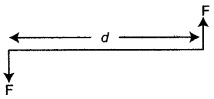Two equal parallel and opposite forces acting on a rigid body at different points constitute a couple. The distance ‘d’ is called arm of the couple.

Question 2: Is it possible to have an accelerated motion with a constant speed? Explain.Yes, it is possible to have accelerated motion with constant speed, when a body is moving in a circular path. The body changes its path so there is a change in direction (velocity) but its speed remains the same.

Question 3: One end of a spring is kept fixed while the other end is stretched by a force as shown in the diagram.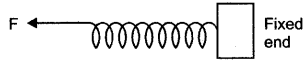(i) Copy the diagram and mark on it the direction of the restoring force.
(ii) Name one instrument which works on the above principle.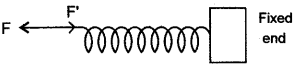(i) F’ in the fig. shows the direction of restoring force.
(ii) Spring balance.

Question 4: A boy of mass 30 kg is fitting at a distance of 2 m from the middle of a see-saw. Where should a boy of mass 40 kg sit so as to balance the see-saw?
Solution: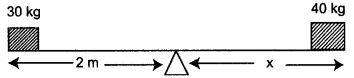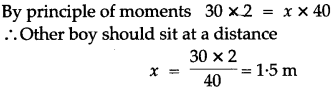Question 5: Write the expression for calculating the moment of force about a given point.Moment of force about the point O
= Force × Perpendicular distance of force from the point O
= F × OP

Question 6: The moment of a force of 10 N about a fixed point O is 5 Nm. Calculate the distance of the point O from the line of action of the force.
Solution: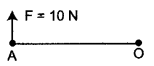Given: Moment of force = 5 Nm
Force = 10 N
According to the formula,
Moment of force = F × OA
5 Nm = 10 × OA
OA = 5/10
= 0.5 m

Question 7: A boy sits and stands repeatedly. Draw a graph showing the variation of potential energy with time.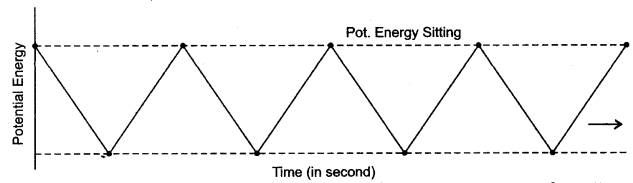Question 8: A ball is placed on a compressed spring. When the spring is released, the ball is observed to fly away.
(i) What form of energy does the compressed spring possess?
(ii) Why does the ball fly away?
Answer: (i) Compressed spring possess potential energy.
(ii) Potential energy of the spring is imparted to the ball in the form of kinetic energy.

Question 9: Draw a diagram to show the energy changes in an oscillating simple pendulum. Indicate in your diagram how the total mechanical energy in it remains constant during the oscillation.
Answer: Diagram shows a freely oscillating pendulum.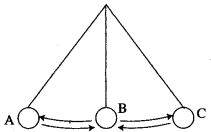In position A, it has max. P.E. and zero K.E.
In position B, it has zero P.E. and max. K.E.
In position C, it has max. P.E. and zero K. E.
Thus, experiment clearly proves that sum of total of energy is conserved, but it can change its form.

Question 10: What is the form of that graph between
(i) K.E. and velocity for a constant mass?
(ii) K.E. and mass for a constant velocity?
Answer: (i) We have, for a constant mass,
K.E. ∝ v2
Hence, the graph is a parabola.(ii) We have, for a constant velocityK.E. ∝ m
Hence, the graph is a straight line.

Question 11: Define an Inclined plane.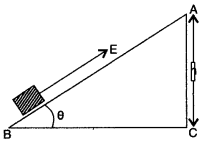An inclined plane is usually a smooth, flat rigid surface inclined at an angle (θ) to the horizontal. It is used to raise heavy loads with relatively small force. The longer the slope, the smaller is the effort needed.

Question 1: Two unlike forces (parallel) of 10 N and 25 N act at a distance of 12 cm. from each other. Find the point about which the body balance.
Solution: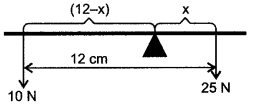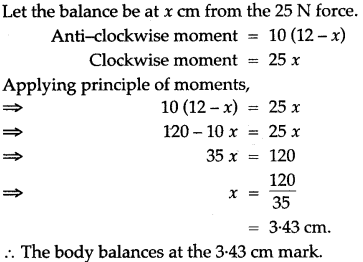Question 2: A uniform metre scale is balanced at 40 cm mark, when weights of 20 gf and 5 gf are suspended at 5 cm mark and 75 cm mark respectively. Calculate weight of metre scale.
Solution: A metre scale is uniform, therefore its weight acts at 50 cm mark.
Taking moments about to cm mark.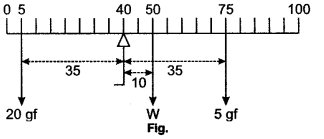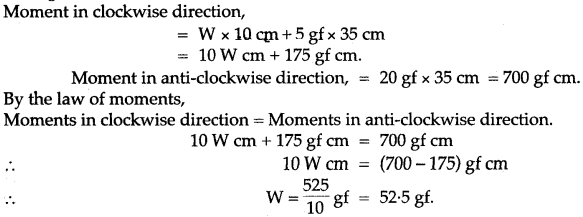Question 3: Give an experiment to verify the principle of moments.
Answer: Verification of Principle of Moments:
Suspend a metre scale horizontally its mid point O’, by means of a thread with its other end connected to a fixed support.
Now suspend some weights on both sides of the mid-point and adjust their distances in such a way that scale again becomes horizontal.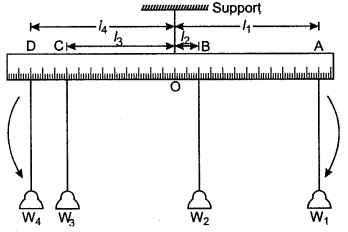Let W1, W2 and W3, W4 be the weights suspended and l1, l2 and l3, l4 are respective lengths.
The weights W1 and W2 tend to turn the scale clockwise while the weights W3 and W4 tend to turn the scale anti-clockwise.
Total clockwise moments = W1 × l1 + W2 × l2
Total anti-clockwise moments = W3 × l3 + W4 × l4
In equilibrium, when the scale is horizontal, it is found that:
Total clockwise moments = Total anti-clockwise moments
W1l1 + W2l2 = W3l3 + W4l4
This verifies the principle of moments.

Question 4: A uniform metre rule rests horizontally on a knife-edge at the 60 cm mark when a mass of 10 gram is suspended from one end. At which end must this mass be suspended? What is the mass of the rule?
Solution: Let M gram be the mass of the rule. Since density of the rule is uniform, its weight Mg will act at its middle point i.e., at the 50 cm mark (Fig.).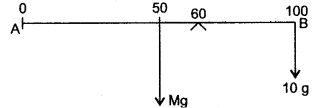The weight of the rule produces an anti-clockwise moment about the knife-edge O. In order to balance it, 10 gram mass is to be suspended at the end B, to produce a clockwise moment.
From the principle of moments,
Anti-clockwise moment = Clockwise moment
Mg × (60 – 50) = 10 g × (100-60)
or Mg × 10 = 10 g × 40
∴ M = 40 gram

Question 5: A uniform metre scale can be balanced at the 70.0 cm mark when a mass of 0.05 kg is hung from the 94.0 cm mark.
(i) Draw a diagram of the arrangement.
(ii) Find the mass of the metre scale.
Solution: (i) Diagram of the given arrangement is shown below.(ii) As the given meter scale is a uniform scale. So its centre of gravity lies at 50 cm. Let mass of meter scale be W1 kg.
By principle of moments,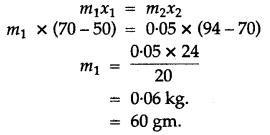Question 6: A block of mass 30 kg is pulled up a slope, as shown in diagram with a constant speed, by applying a force of 200 N parallel to slope.
A and B are initial and final positions of block.(i) Calculate the work done by force in moving the block from A to B.
(ii) Calculate P.E. gained by block. [g = 10ms-2]
Solution: (i) Work done in moving the block from = F × d
A to B = 200 N × 3m = 600 J
(ii) Gain in potential energy = mgh = 30 × 10 × 1.5 = 450 J.

Question 7: (i) A 200g ball is thrown vertically upward with a initial velocity of 30 ms-1. Draw a velocity time graph for the motion of ball.
(ii) How long will the ball take to reach the highest point?
(iii) What will be the kinetic energy of ball, when it returns to starting point, neglecting the air resistance?
(iv) What will be the potential energy of ball at highest point?
Solution: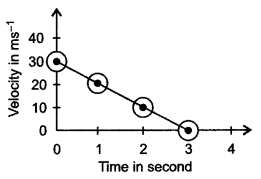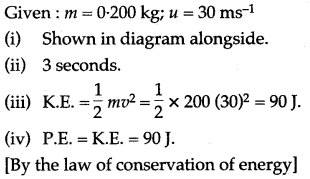Question 8: A truck driver can load oil drums into the back of the truck by pushing them up a sloping plank, or by lifting them directly. Each drum has a mass of 80 kg, the plank is 3 m long, and the back of the truck is 0.8 m above the ground.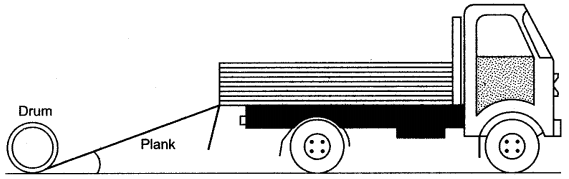(i) How much force would be needed to lift a drum into the truck directly/without using the plank? (Take g = 10 m/s2)
(ii) How much energy would be required in lifting the drum into the truck without the plank?
(iii) If the force needed to push the drum up the plank is 300 N, why is this less than the answer to part (i)?
(iv) When the truck is loaded, the driver drives off. List the major energy changes that take place in moving the truck.
(v) The driver has to stop at the factory gates. What happens to the kinetic energy of the truck?
Solution: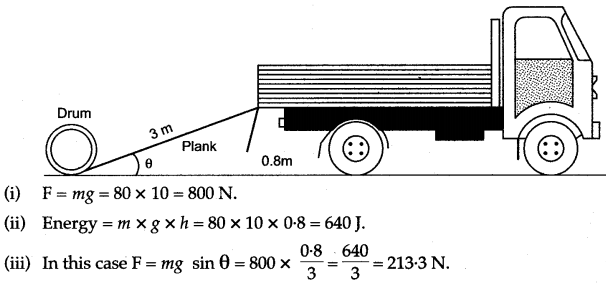It is less than the answer in part (i) as the efforts acts through 3 m whereas the weight is lifted up through 0.8 m. The effort needed may be 300 N instead of 213.3 N owing to loss of energy in overcoming friction and air resistance.
(iv) The energy released owing to combustion of fuel changes into kinetic energy of the truck and load, and this changes in heat energy of the pebbles on the road and of wheels.
(v) The kinetic energy is dissipated as heat.

Question 9: Show that in case of a body falling freely under gravity, total mechanical energy remains conserved (neglect air resistance).
Answer: Let a body of mass m fall freely under gravity from height h above ground.
Let A, B and C be the positions of body.
Let x be the distance fallen from A to B.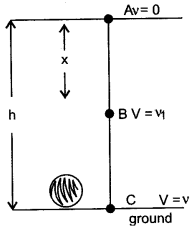From (i), (ii) and (iii) it is clear that sum of mechanical energy remains same at any point in the path of free fall of a body.

Question 10: What is nuclear energy? Explain the principle of producing electricity using the nuclear energy is a nuclear reactor.
Answer: The process whereby an atomic nucleus breaks up into two or more major fragments with the release of tremendous amount energy is called nuclear fusion and the energy thus released is nuclear energy.
Principle: The production of electricity using nuclear energy is based on principle in which steam is produced by heating water through heat energy released during the controlled nuclear fission of uranium-235 (or plutonium-239). When atoms of uranium fuel are hit by neutrons they fission (split), releasing heat and more neutrons. Under controlled conditions, these other neutrons can strike more uranium atoms, splitting more atoms, and so on. Thereby, continuous fission can take place, forming a chain reaction releasing heat. The heat is used to turn water into steam that, it turn, spins a turbine that generates electricity. At present nuclear power is used to generate 3% of all the country’s electricity.### Short Numericals

Question 1: Derive a relation between the S.I. and C.G.S. unit of force.
Solution: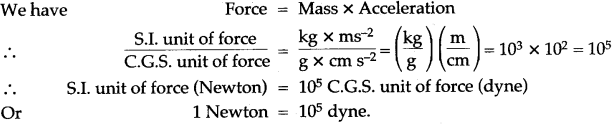Question 2: A man can open a nut by applying a force of 150 N by using a lever handle of length 0.4 m. What should be the length of the handle if he is able to open it by applying a force of 60 N?
Solution: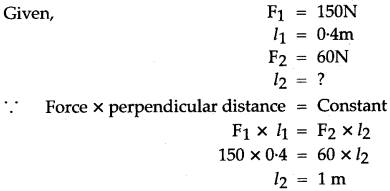Question 3: The perpendicular distance between the point of application of force and turning point is 1.75 m, when a force of 80 N acts on a rigid body. Calculate the moment of force.
Solution:Question 4: Calculate the minimum distance between point of application of force and axis of rotation of a rigid body, if a force 125 N produces a moment of force of 25.75 Nm.
Solution: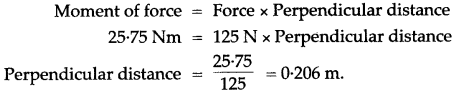Question 5: A couple of 15 N force acts on a rigid body, such that arm of couple is 85 cm. Calculate moment of couple in SI system.
Solution: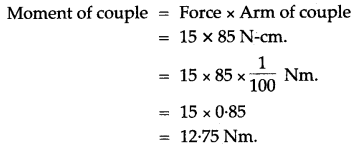Question 6: Calculate magnitude of the force of a couple, when arm of couple is 63 cm and moment of couple is 21 Nm.
Solution:Question 7: Calculate the change in the Kinetic energy of a moving body if its velocity is reduced to 1/3rd of the initial velocity.
Solution:Question 8: If the power of a motor is 40KW, at what speed can it, raise a load of 20,000 N?
Solution: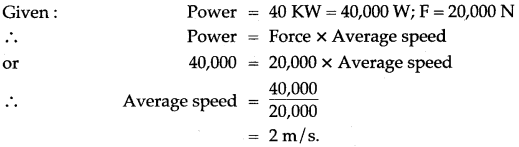Question 9: Calculate the length of arm of couple, if a force of 13 N produces a moment of couple of 14.3 Nm.
Solution:Question 10: Calculate the work done when:
(i) A 5 kg weight is lifted 10 m vertically. (g = 9.8 m/s2)
(ii) A car is moved on a rough road through 30 m against a frictional resistance of 75 N.
Solution:Question 11: A coolie carrying a load on his head and moving on a frictionless horizontal platform does no work. Explain the reason why.
Solution:Question 12: A girl of mass 35 kg climbs up from the first floor of a building at a height 4 m above the ground to the third floor at a height 12 m above the ground. What will be the increase in her gravitational potential energy? [g = 10 ms-2]
Solution: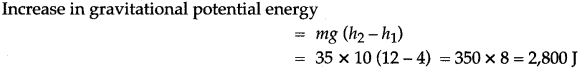Question 13: A force is applied on a body of mass 20 kg moving with a velocity of 40 ms-1. The body attains a velocity of 50 ms-1 in 2 seconds. Calculate the work done by the body.
Solution:Question 14: (i) The world record for weight lifting was held by Sergei Didyk of USSR. He lifted 261 kg to a height of 2.3 m in 4s; find:
(a) Weight lifted by him (b) Work done by him
(c) Power developed by him. (g = 10m/s2)
(ii) The work done by the heart is 1 Joule per beat. Calculate the power of the heart if it beats 72 times in one
Solution:Question 15: How long should an electric motor, of power 2 H.P. operate, so as to pump 5 m3 of water from a depth of 15m. [Take g = 10 N kg-1]
Solution:Question 16: A man having a box on his head, climbs, up a slope and another man having art identical box walks the same distance on a levelled road. Who does more work against the force of gravity and why?
Solution: Man having a box on his head who climbs up a slope does more work against the force of gravity because he has more potential energy by virtue of his position i.e., height.
As,    P.E. = W.D. = F × S
= mg × h

Question 17: A machine raises a load of 750 N through a height of 16 m in 5 seconds. Calculate the power at which the machine works.
Solution:Question 18: State the C.G.S. unit of work. How it is related to its S.I. unit?
Solution:Question 19: A body of mass 0.2 kg falls from a height of 10 m to a height of 6 m above the ground. Find the loss in potential energy taking place in the body. [g = 10ms-2]
Solution: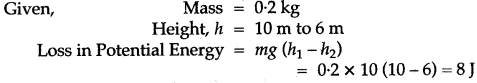Question 20: A ball of mass 200 g falls from a height of 5 m. What will be its kinetic energy when it just reaches the ground? (g = 9.8 m s-2)
Solution:Question 21: Two bodies, A and B of equal mass are kept at heights 20 m and 30 m respectively. Calculate the ratio of their potential energies.
Solution:Question 22: A ball of mass 0.20 kg is thrown vertically upward with an initial velocity of 20 m/s. Calculate the maximum potential energy it gains as it goes up.
Solution: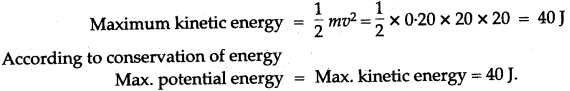Question 23: A body is thrown vertically upwards. Its velocity keeps on decreasing. What happens to its kinetic energy as its velocity becomes zero?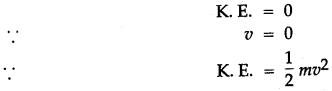Question 24: A moving body weighing 400 N possesses 500 J of kinetic energy. Calculate the velocity with which the body is moving. (g = 10 ms2)
Solution:### Long Numericals

Question 1: How do you define force? What are the forces acting on a man standing in a lift? If the lift moves down with an acceleration of 2 ms-2, what is the net force on the man if his mass is 50 kg?
Solution: Net force acting on a body is equal to mass into acceleration or we may define force as a push or pull which changes or tends to change the state of rest or of uniform motion in a straight line of the body on which it acts.
The forces acting on a man in a lift are
(i) weight of the man acting vertically downwards,
(ii) the reaction of the floor acting vertically upwards.
In a lift accelerating downwards the net force on the man is
F = m(g – a) = 50(9.8 – 2)
= 50 × 7.8 = 390 N.

Question 2: A boy of mass 40 kg runs up a height of 30 steps, each 20 cm high. Find:
(i) The force of gravity acting on the boy.
(ii) The work done by the boy against gravity. (Take g = 9.8 ms-2)
Solution: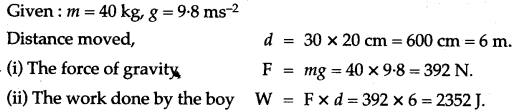Question 3: A coolie is pushing a box weighing 1500 N up an inclined plane 7.5 m long on to a platform, 2.5 m above the ground.
(i) Calculate the mechanical advantage of the inclined plane.
(ii) Calculate the effort applied by the coolie.
(iii) In actual practice, the coolie needs to apply more effort than what is calculated. Give one reason why you think the coolie needs to apply more effort.
Solution: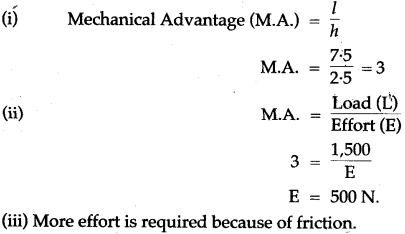Question 4: Calculate the work done when:
(i) A 5 kg weight is lifted 10 m vertically upward (g = 9.8 ms-2).
(ii) A car is moved on a rough road through 3 m against a frictional resistance of 75 N.
Solution:Question 5: An electric motor of power 100 W is used to drive the stirrer in a water bath. If 50% of the energy supplied to the motor is spent in stirring the water. Calculate the work done on the water in one minute.
Solution:Question 6: The weights of two bodies are 2.0 N and 2.0 kgf respectively what is the mass of each body?
Solution: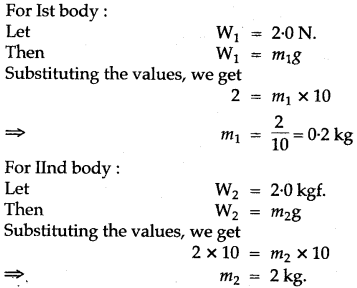Question 7: A truck weighing 1000 kg changes its speed from 36 km/h to 72 km/h in 2 minutes. Calculate the work done by the engine and its power.
Solution: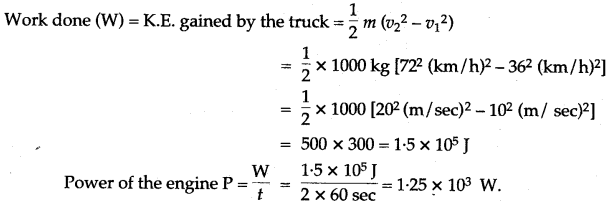Question 8: A coolie carries a load of 30 kgf through a distance of 500 m in 5 minutes while another coolie B carries the same load through the same distance in 10 minutes. Compare the (i) work done, and (ii) power developed. (Take: g = 10md-2)
Solution: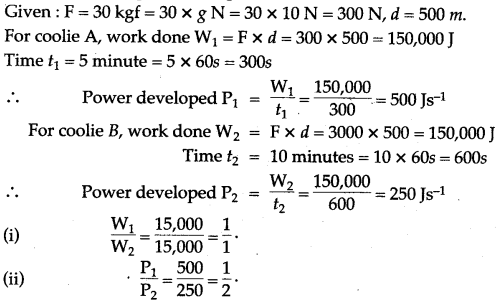Question 9: A girl of mass 40 kg runs a height of 80 stairs, each 25 cm high with a load of 20 kg on her head in 25 sec. If g is 10 m/s2, find:
(i) Gravitational force acting on the girl.
(ii) Work done by her.
(iii) Useful work done by her.
(iv) Her power in watt.
Solution:Question 10: A man weighing 700 N runs up a flight of 15 steps, each 20 cm high, in 5 seconds. Calculate the power of the man.
Solution:Question 11: A water pump raises 50 kg of water through a height of 25 m in 5s. Calculate the power Supplied by the pump (Take: g = 10 N kg-1).
Solution:Question 12: An electric motor pump is 60% efficient and is rated 2.5 H.P. Calculate the maximum load it can lift through a height of 10m in 8 sec. (1 H.P. = 750 W).
Solution:Question 13: For the same kinetic energy of a body, what should be the change in its velocity if its mass is increased nine times?
Solution:Question 14: Calculate the kinetic energy of a body of mass 0.1 kg. and momentum 40kg m/s.
Solution:Question 15: A body of mass 50 kg has a momentum of 3000 kg-1 ms. Calculate:
(j) the kinetic energy of the body.
(ii) the velocity of the body.
Solution: (j) m =50 kg. p = 3000 kg ms-1Question 16: A body of mass m falls from a height h1 to a height h2, above the ground (h1 > h2). What is the loss in potential energy?Question 17: Obtain an expression for the kinetic energy of a body of mass m moving with a velocity v?
Answer: Let the body is brought to rest by a retarding force F after travelling a distance s.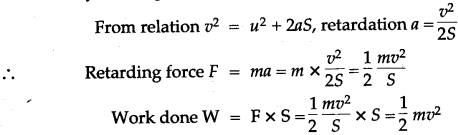Hence K.E = ½ mv2

Question 18: A body of mass m is taken from a height h to 2h. What is the increase in its potential energy?
Initial potential energy = mgh
Final potential energy = mg × 2h = 2mgh
Increase in potential energy = Final potential energy – Initial potential energy
= 2mgh – mgh = mgh.

Question 19: A ball of mass 50 g falls from a height of 2m and rebounds from the ground to 1.6 m. Find:
(i) The potential energy possessed by the ball when initially at rest.
(ii) The kinetic energy of the ball before it hits the ground.
(iii) The final potential energy of the ball.
(iv) The loss in kinetic energy of the ball on collision. (Take: g = 10N kg-1)
Solution:Question 20: In a hydroelectric power station, 1000 kg of water is allowed to drop through a height of loo m in 1 sec. If the conversion of potential energy to electric energy is 60%. Calculate the power output. [Take: g = 10m/sec2]
Solution: In a hydroelectric power station:
Mass of water (m) 1000 kg.
Height (h) = 100 m.
Time (t) = ¡sec.
Produced potential energy (P.E.) = mgh = 1000 × 10 × 100 JouIe
= 1000000 joule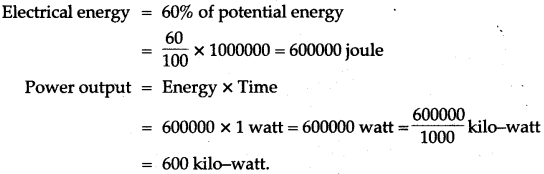Question 21: On’a see-saw, two children of masses 30 kg and 50 kg are sitting on one side of it at distance 2 m and 2.5 m respectively, from its middle, where should a man of mass 74 kg sit to balance it?
Solution: Let the two children be sitting on the left arm. They will produce anti-clockwise moment due to their weights about the middle point.
Total Anti-clockwise moment = 30 kgf × 2 m + 50 kgf × 2.5 m
= 60 kgf m + 125 kgf m = 185 kgf m
To balance it, the man should sit on the right arm (to produce clockwise moment). Let his distance from the middle be x m. ThenQuestion 22: A force of 1 kgf displaces a body by a distance of 10 cm (i) in direction of force, (ii) normal to the force (iii) at an angle of 60° to the direction of force. Calculate the amount of work done in each case. (Take: g = 9.8 ms-2 )
Solution: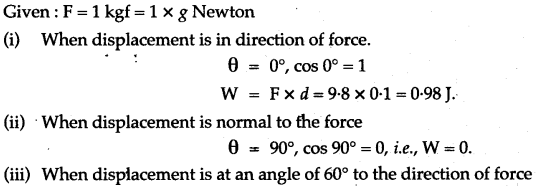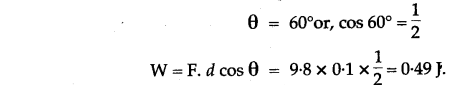For More Resources